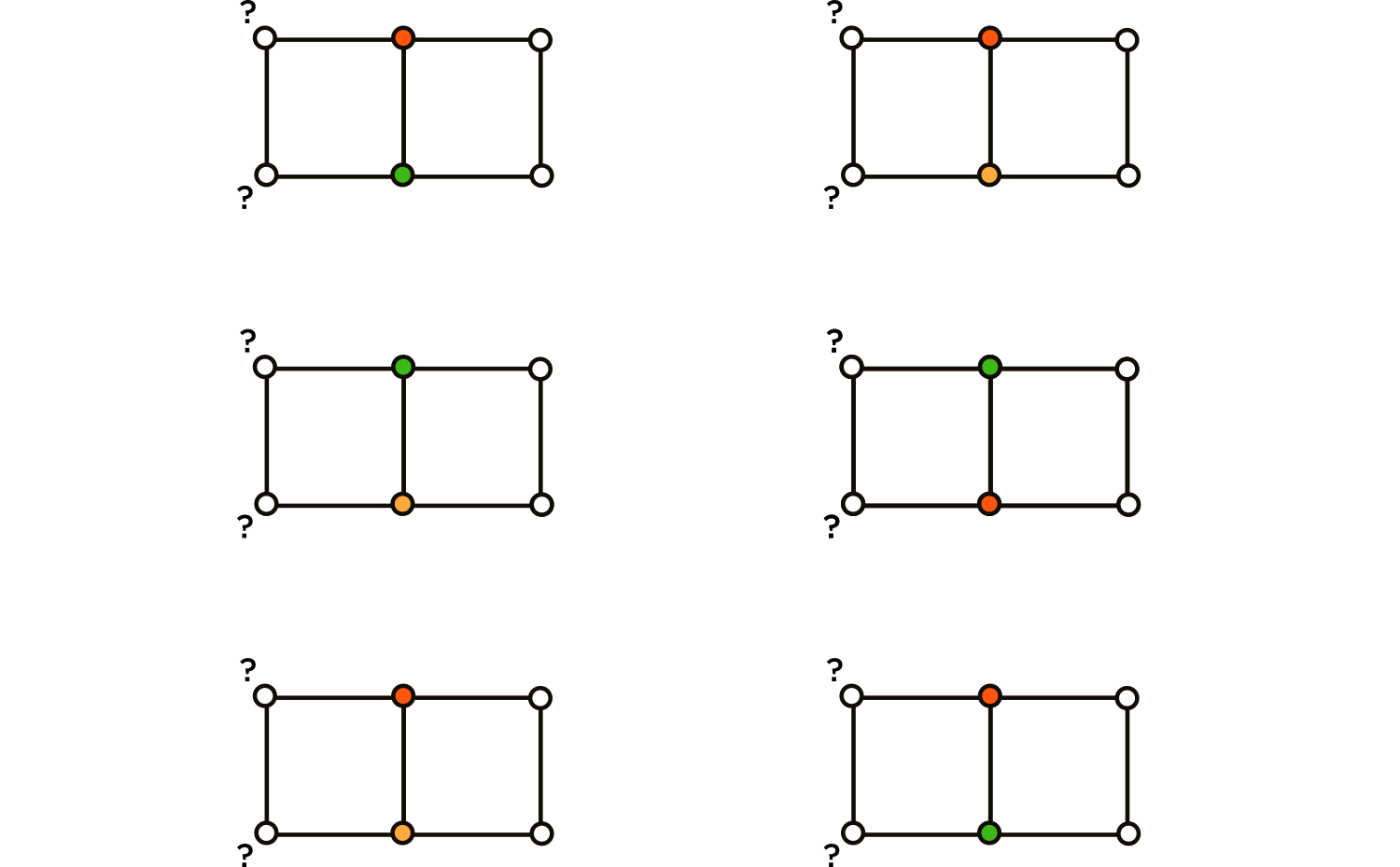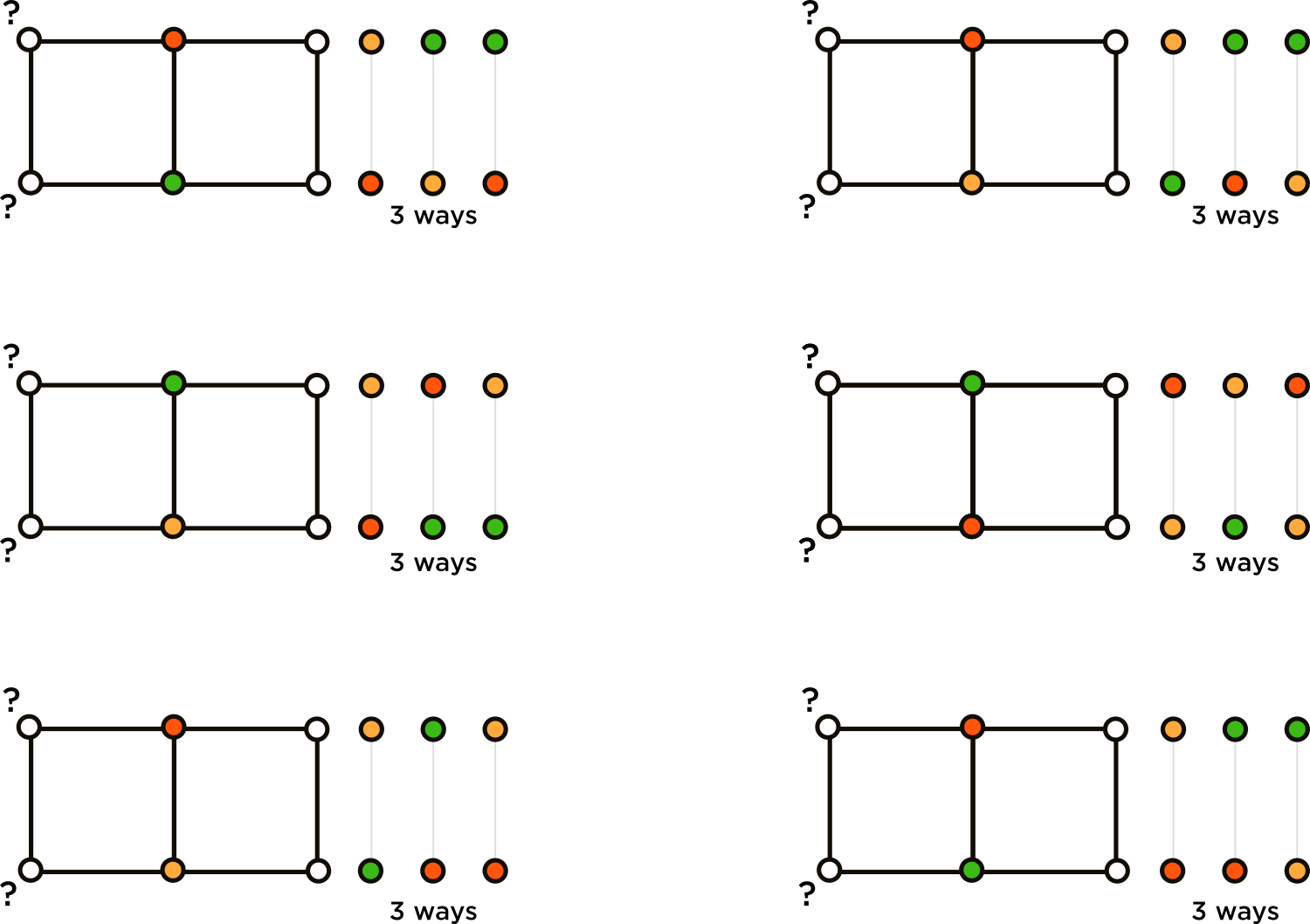# How do you get 18÷(6×3) = 3 different ways to color the right-hand square given the colors of the two vertices both squares share?

• Module 3 Week 3 Day 12 Challenge Part 5 Mini-Question

Explanation:
There are $$\frac{18}{3 \times 2}=3$$ different ways to color the right-hand square given the colors of the two vertices both squares share, because there are $$2 \times 3=6$$ different color orders which the two vertices on the shared side could have. So, $$\frac{1}{6}$$ of the $$18$$ different squares should contain the exact color order on their left side that the right-hand square has on its right side. Therefore, there are $$18 \times 3=54$$ different ways in which we could color the diagram, such that no two adjacent vertices are the same color.

How do you get $$\frac{18}{3 \times 2}?$$

• @spaceblastxy1428 Hi again!In the Day 12 Challenge Part 4 Mini-Question, we saw that there were $$18$$ ways to color the right-hand square.

@spaceblastxy1428 said in Question:

Explanation:
There are $$\frac{18}{3 \times 2}=3$$ different ways to color the right-hand square given the colors of the two vertices both squares share, because there are $$2 \times 3=6$$ different color orders which the two vertices on the shared side could have.

How do you get $$\frac{18}{3 \times 2}?$$

The sentence is saying that these $$18$$ ways come from $$6 \times 3,$$ where $$6$$ is the number of ways to color the middle two vertices and $$3$$ is the ways to color the other two vertices of the square given that the two middle are colored. The phrase given indicates that we are fixing the colors of the middle two vertices. Then there are $$3$$ ways to color the other two vertices.

Another way is to think of the $$18$$ ways as being in $$6$$ groups based on what the coloring of the middle two vertices is. There are $$6$$ ways to color the middle two vertices.Each of these $$6$$ ways is like a group of $$3,$$ as there are $$3$$ ways to color the other two vertices of the square.This is all that the sentence is saying, that there are $$\frac{18 \text{ total ways to color the square}}{6 \text{ groups }} = 3 \text{ ways per group}.$$

Thank you for asking this question! Module 3 is so much fun to learn, because of all the new and creative tricks that come up. I hope you are enjoying it too.